Operations on Complex Numbers (page 2 of 3)

Sections: Introduction, Operations with complexes, The Quadratic FormulaComplex numbers are "binomials" of a sort, and are added, subtracted, and multiplied in a similar way. (Division, which is further down the page, is a bit different.) First, though, you'll probably be asked to demonstrate that you understand the definition of complex numbers.

• Solve 3 – 4i = x + yi
• Finding the answer to this involves nothing more than knowing that two complex numbers can be equal only if their real and imaginary parts are equal. In other words, 3 = x and –4 = y.

To simplify complex-valued expressions, you combine "like" terms and apply the various other methods you learned for working with polynomials.

• Simplify (2 + 3i) + (1 – 6i).

(2 + 3i) + (1 – 6i) = (2 + 1) + (3i – 6i) = 3 + (–3i) = 3 – 3i

• Simplify (5 – 2i) – (–4 – i).

(5 – 2i) – (–4 – i)

= (5 – 2i) – 1(–4 – i) = 5 – 2i – 1(–4) – 1(–i)

= 5 – 2i + 4 + i= (5 + 4) + (–2i + i)

= (9) + (–1i) = 9 – i

You may find it helpful to insert the "1" in front of the second set of parentheses (highlighted in red above) so you can better keep track of the "minus" being multiplied through the parentheses.

• Simplify (2 – i)(3 + 4i).

(2 – i)(3 + 4i) = (2)(3) + (2)(4i) + (–i)(3) + (–i)(4i)

= 6 + 8i – 3i – 4i2 = 6 + 5i – 4(–1)

= 6 + 5i + 4 = 10 + 5i

For the last example above, FOILing works for this kind of multiplication, if you learned that method. But whatever method you use, remember that multiplying and adding with complexes works just like multiplying and adding polynomials, except that, while x2 is just x2, i2 is –1. You can use the exact same techniques for simplifying complex-number expressions as you do for polynomial expressions, but you can simplify even further with complexes because i2 reduces to the number –1.

Adding and multiplying complexes isn't too bad. It's when you work with fractions (that is, with division) that things turn ugly. Most of the reason for this ugliness is actually arbitrary. Remember back in elementary school, when you first learned fractions? Your teacher would get her panties in a wad if you used "improper" fractions. For instance, you couldn't say " 3/2 "; you had to convert it to "1 1/2". But now that you're in algebra, nobody cares, and you've probably noticed that "improper" fractions are often more useful than "mixed" numbers. The issue with complex numbers is that your professor will get his boxers in a bunch if you leave imaginaries in the denominator. So how do you handle this?

• Simplify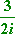This is pretty "simple", but they want me to get rid of that i underneath, in the denominator. The 2 in the denominator is fine, but the i has got to go. To do this, I will use the fact that i2 = –1. If I multiply the fraction, top and bottom, by i, then the i underneath will vanish in a puff of negativity: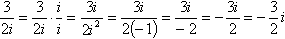So the answer is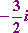This was simple enough, but what if they give you something more complicated?

• Simplify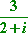If I multiply this fraction, top and bottom, by i, I'll get: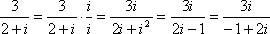Since I still have an i underneath, this didn't help much. So how do I handle this simplification? I use something called "conjugates". The conjugate of a complex number a + bi is the same number, but with the opposite sign in the middle: a – bi. When you multiply conjugates, you are, in effect, multiplying to create something in the pattern of a difference of squares:Note that the i's disappeared, and the final result was a sum of squares. This is what the conjugate is for, and here's how it is used: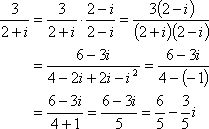So the answer is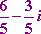In the last step, note how the fraction was split into two pieces. This is because, technically speaking, a complex number is in two parts, the real part and the i part. They aren't supposed to "share" the denominator. To be sure your answer is completely correct, split the complex-valued fraction into its two separate terms.

<< Previous  Top  |  1 | 2 | 3  |  Return to Index  Next >>

 Cite this article as: Stapel, Elizabeth. "Operations on Complex Numbers." Purplemath. Available from     https://www.purplemath.com/modules/complex2.htm. Accessed [Date] [Month] 2016

MathHelp.com Courses